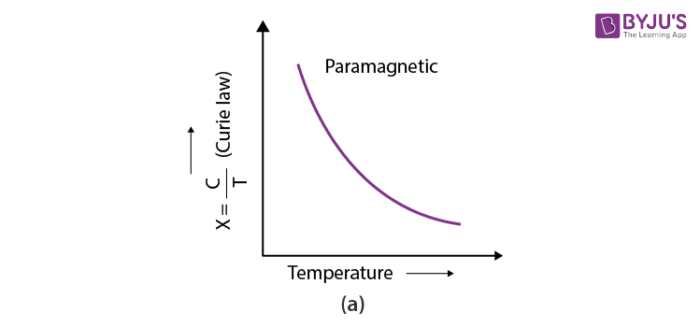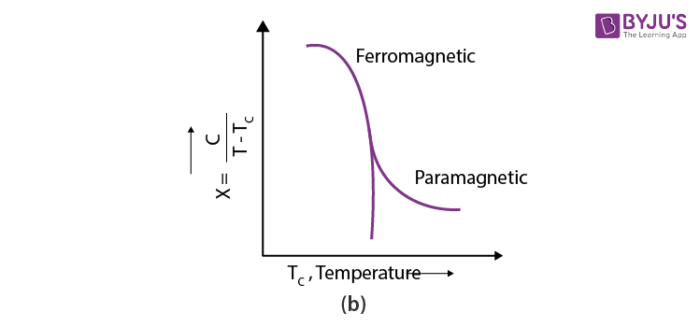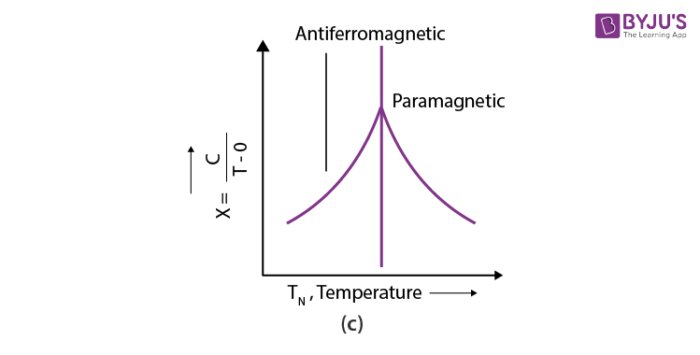# A curve between magnetic moment and temperature of magnet is

The magnetic moment is inversely proportional to temperatureThe magnetism of a magnet falls with rising temperature and becomes practically zero above curie temperature.

### Graphs showing the variation of magnetic properties on changing temperature:

In paramagnetic material, with the increase in the magnetic field, the magnetization of the material increases. When the material is heated the magnetization starts decreasing, so the magnetization of the material is inversely proportional to temperature. This relationship is known as Curie’s law.

M = C×(B/T)

Where, M = magnetization of the material

C= Curie’s constant

B= applied magnetic field

T= Temperature(0)(0)